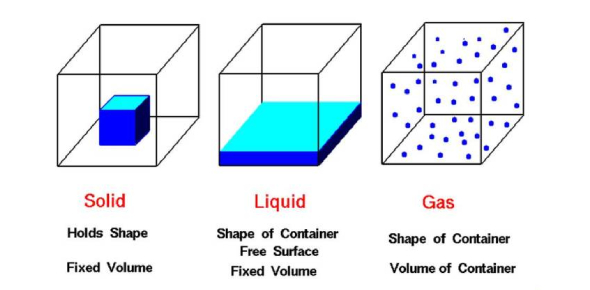# Practice Test: Matter And Its Properties Quiz!

20 Questions | Total Attempts: 8171SettingsThis quiz has been created for students to test their knowledge and understanding of the concepts involved with matter including states of matter, changes in matter, what is matter, what is mass, volume, density, etc. There is a list of 20 questions. Read them carefully and answer. So, let's try out the quiz All the best!

• 1.
The density of an object is a ---
• A.

Chemical Property

• B.

Combustible Property

• C.

Physical Property

• D.

Reactive Property

• 2.
A pure substance always has ______ desisty when measured under _____ conditions.
• A.

The same; different

• B.

The same; the same

• C.

A different; the same

• D.

A different; different

• 3.
A solution is a type of--
• A.

Matter

• B.

Mixture

• C.

Balance

• D.

Combustibility

• 4.
Weight is measured on a scale. Mass is measured on a --
• A.

Sale

• B.

Barometer

• C.

Balance

• D.

Thermometer

• 5.
Which of the following does Not signal a chemical change?
• A.

Change in state

• B.

Production of gas

• C.

Color change

• D.

Production of light and heat

• 6.
Making skim milk is an example of ---
• A.

Combustibility

• B.

Solubility

• C.

Using physical properties to separate a mixture

• D.

Using chemical properties to seperate a mixture

• 7.
A person's _____ is different on a high mountain than it is at sea level.
• A.

Chemical property

• B.

Density

• C.

Mass

• D.

Weight

• 8.
Two similar looking substances are burned. One produces a red flame, and the other produces a yellow flame. This is an example of ---
• A.

A flame test

• B.

An indicator test

• C.

A combustibility test

• D.

A reactivity test

• 9.
Given a solution of sugar and water, and water and pepper, which is true.
• A.

If stired, the pepper would have the same solubility as sugar.

• B.

Pepper is soluble in water, bur sugar is not.

• C.

Sugar is soluble in water, but pepper is not.

• D.

Sugar and peper have the same solubility

• 10.
Other ____of substance, like color, size, and hardness, can be found without changing the substance into something else.
• A.

Reactivity

• B.

Physical properties

• C.

Evaporation

• D.

Chemical Properties

• 11.
An object's ____ is the pull of gravity on the object
• A.

Solubility

• B.

Mass

• C.

Weight

• D.

Volume

• E.

Matter

• 12.
A ____ has a definate volume but no definite shape.
• A.

Solid

• B.

Liquid

• C.

Gas

• D.

Matter

• E.

Density

• 13.
A ____ does not have a definite volume or a definite shape.
• A.

Gas

• B.

Solid

• C.

Liquid

• D.

Volume

• E.

Weight

• 14.
A _____ has both definite volume and shape.
• A.

Liquid

• B.

Solid

• C.

Weight

• D.

Gas

• E.

Matter

• 15.
You can divide the mass of an object by its volume to find the object's ______.
• A.

Physical properties

• B.

Solubility

• C.

Reactivity

• D.

Density

• E.

Volume

• 16.
All material that takes up space is called.
• A.

Mass

• B.

Matter

• C.

Solubility

• D.

Weight

• E.

Volume

• 17.
The amount of material in an object is called the object's _____.
• A.

Physical properties

• B.

Density

• C.

Volume

• D.

Weight

• E.

Mass

• 18.
You can measure the amount of space a solid object takes up, or the _____ of a solid object, by placing the object in a graduated cylinder with water and measuring how much water is displaced.
• A.

Mass

• B.

Density

• C.

Volume

• D.

Weight

• E.

Solubility

• 19.
Chemical changes can turn substances into other substances. The ability of a substance to go through a chemical change is called _____.
• A.

Evaporation

• B.

Physical properties

• C.

Combustibility

• D.

Reactivity

• E.

Condensation

• 20.
Sometimes the ability to be dissolved, or ________, can be used to identify a substance.
• A.

Solubility

• B.

Matter

• C.

Volume

• D.

Liquid

• E.

Gas

Related TopicsBack to top